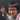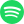# Many-to-Many "Functions"

May 19, 2020 · 1:40 am · Math

One of the things in math that has always irked me a bit is the inability to express the points on a circle elegantly using a function. For example, $x^2 + y^2 = 1$ is an equation for points on a circle centered at $(0,0)$ with a radius of $1$, but if we wanted to express that in a $y=f(x)$ form, there isn’t a great way to do this. You’d be stuck with two functions:

\begin{aligned} y_1 &= \sqrt{1-x^2} \\ y_2 &= -\sqrt{1-x^2} \\ \end{aligned}

In math class, you learn that a function is something that takes a number for an input, does some math on the number, and finally outputs an answer. And typically in English, “functions” as a thing imply a many-to-1 relation, typically denoted $n:1$; that is, any input will always yield a single, numerical answer.

## Always a single answer, you say?

What if $f(x) = \frac{1}{x}$? Then $f(0)$ is $\text{undefined}$, which shouldn’t count as a single answer, right?

That’s right. $\text{undefined}$ doesn’t count as an answer, because it’s a placeholder we use to convey the idea that there isn’t an answer. So are functions actually many-to-0-or-1?

Well, not really, thanks to Domains. But to talk about why, we need to first talk about function mapping.

## Domain and Range

Sidenote:
The term range is actually ambiguous in mathematics. In literature, range has been used in the past to denote both the images and codomain of functions. In high school, when we say range in the context of “Domain and Range”, we’re actually talking about images, and since we don’t really use codomains, there are no issues in practice. But to avoid confusion, I’ll use image in place of range.

We denote the domain and codomain of functions as

$f\colon X \rightarrow Y,$

where $X$ is the domain and $Y$ is the codomain. Recall that the domain is the set of values that are valid/allowed to be plugged into the function, i.e. the function is well-defined only for inputs in the domain. For example, for the function $f(x) = \frac{1}{x}$, the domain is set to

$\{x \in \mathbb{R} \mid x \neq 0\},$

the set of all reals except zero. Which means that $0$ isn’t even a valid input for $f(x) = \frac{1}{x}$. In face, when we say that the relationship between inputs and outputs of a function are $n:1$, the $n$ refers to members in the domain, and the $1$ refers to members in the image. Indeed, functions are, in fact, strictly many-to-1.

## Many-to-Many

Ok so back to the original problem: expressing something like $x^2 + y^2 = 1$, or a $n:m$ relation as a “function”. Where do we begin?

Introducing: binary relations. Binary relations are similar to functions, but instead of using an input-to-output model of thinking, both of the “input” and “output” are used as arguments. In fact, a binary relation $R$ can be considered the set of pairs $(x,y)$ that makes the expression $xRy$ true. The weird $xRy$ notation can be thought of as just a “function” $R$ that takes $x$ and $y$ as inputs and outputs “true” or “false”, also known as a predicate.

“Domains” and “codomains” from functions have an analogy for binary relations too. In English, binary relations are usually instantiated by the following phrase:

A binary relation $R$ over the sets $X$ and $Y$

which creates a set $R$ whose elements are ordered pairs from $X \times Y$, where ”$\times$” is the cartesian product. This makes $R$ a subset of $X \times Y$, expressed as

$R \subseteq X \times Y$

In formal notation, we can also define the relation by $R = \{(x,y) \mid xRy \}$, replacing $xRy$ with a predicate expression. For example, we can define a binary relation

$C = \{(x,y) \mid x^2 + y^2 = 1\}$

over the set $\mathbb{R} \times \mathbb{R}$. Then, we can say $(\sqrt{2},\sqrt{2}) \in C$ and $(\sqrt{2},-\sqrt{2}) \in C$. We finally have something that represents a complete circle, rather than a semicircle with open ends.Streaming direct thought dumps from Yuto Nishida.
Connect with me on LinkedIn! I'm currently listening to: